Your browser doesn't support the features required by impress.js, so you are presented with a simplified version of this presentation.

For the best experience please use the latest Chrome, Safari or Firefox browser.

Rendering Hypercomplex Fractals

Anthony Atella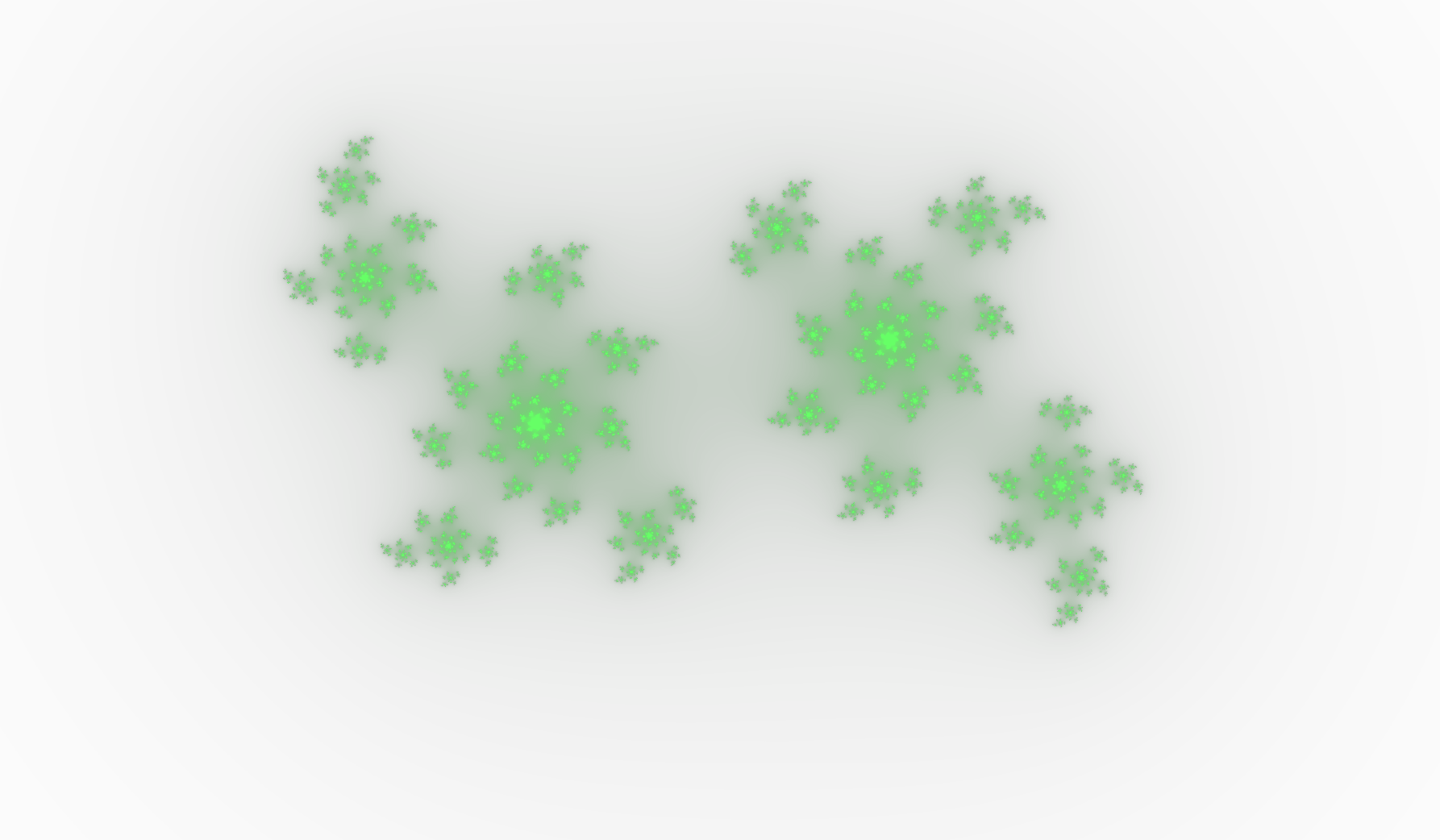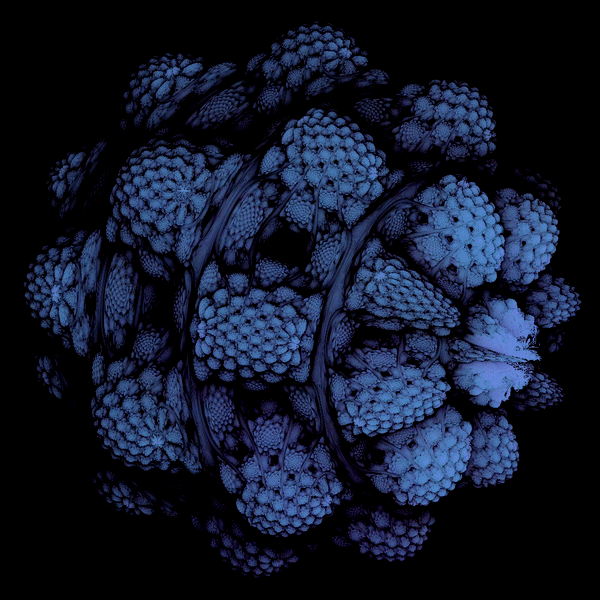Fractals are:

• Mathematical constructs

• Geometric structures

• Statistically self-similar at all scales

• Infinite

Applications:

• Generate 3D topology

• Multi-frequency antennas

• Biological & ecological surveys

• Artwork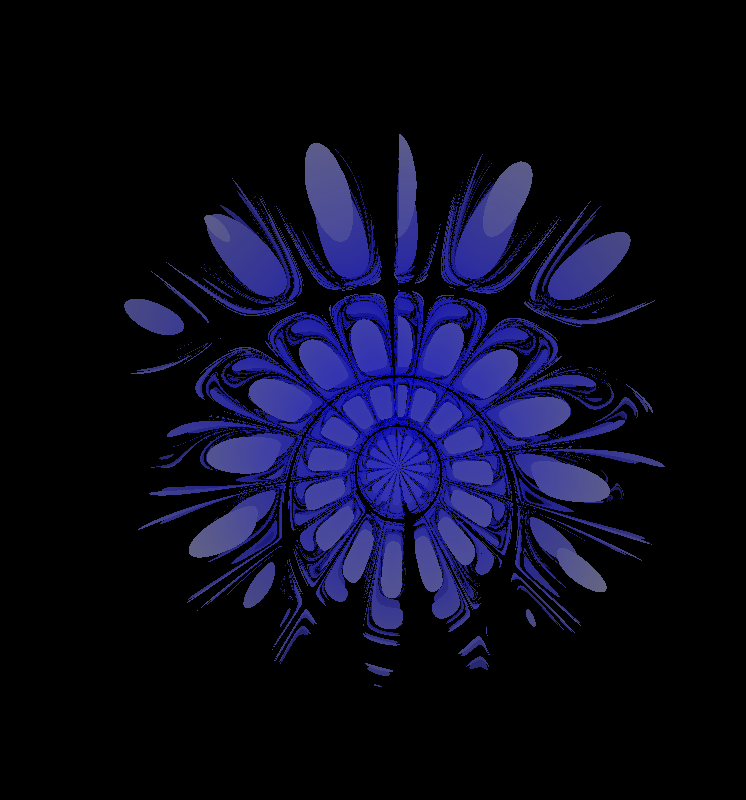Chaos is:

• A fractal rendering
application

• Cross-platform

• Extensible API

Chaos can:

• Edit and preview fractals

• Export .png & .mp4 files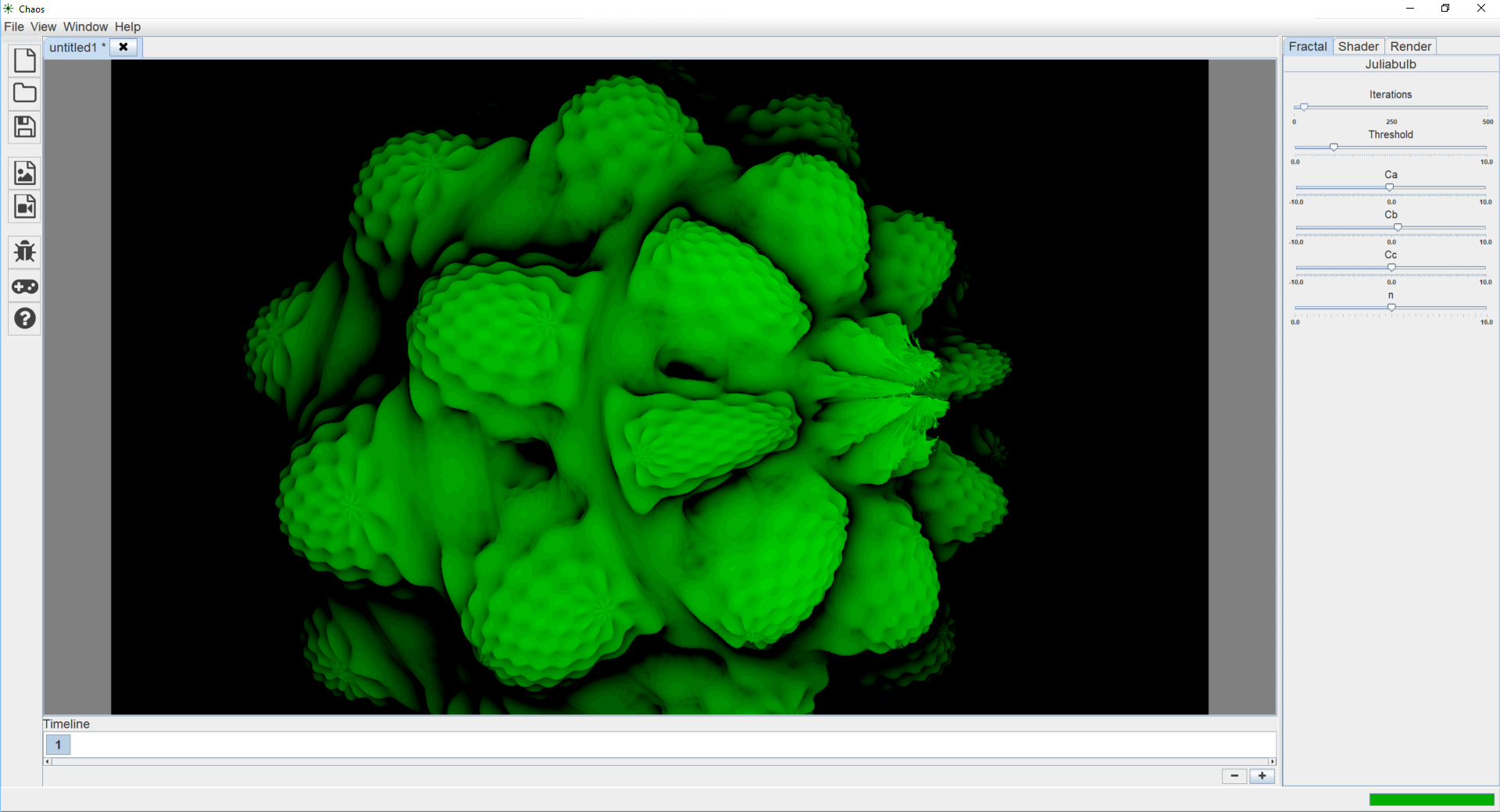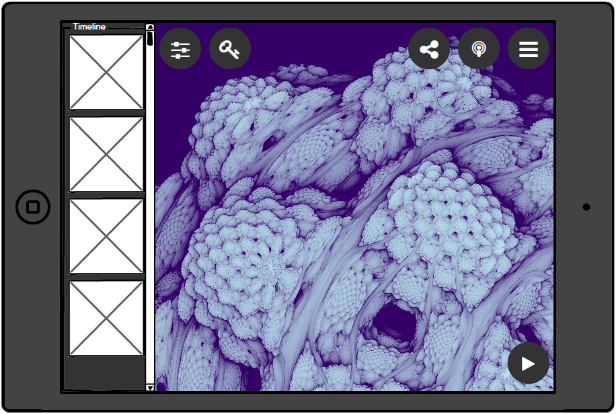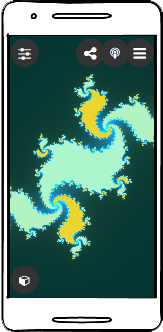Thesis:

• Fractal rendering can be
broken into steps

• Steps should be abstracted
from each other

• Provides maximum extensibility
and reusability

Rendering Steps:

• Fractal Calculation

• Rendering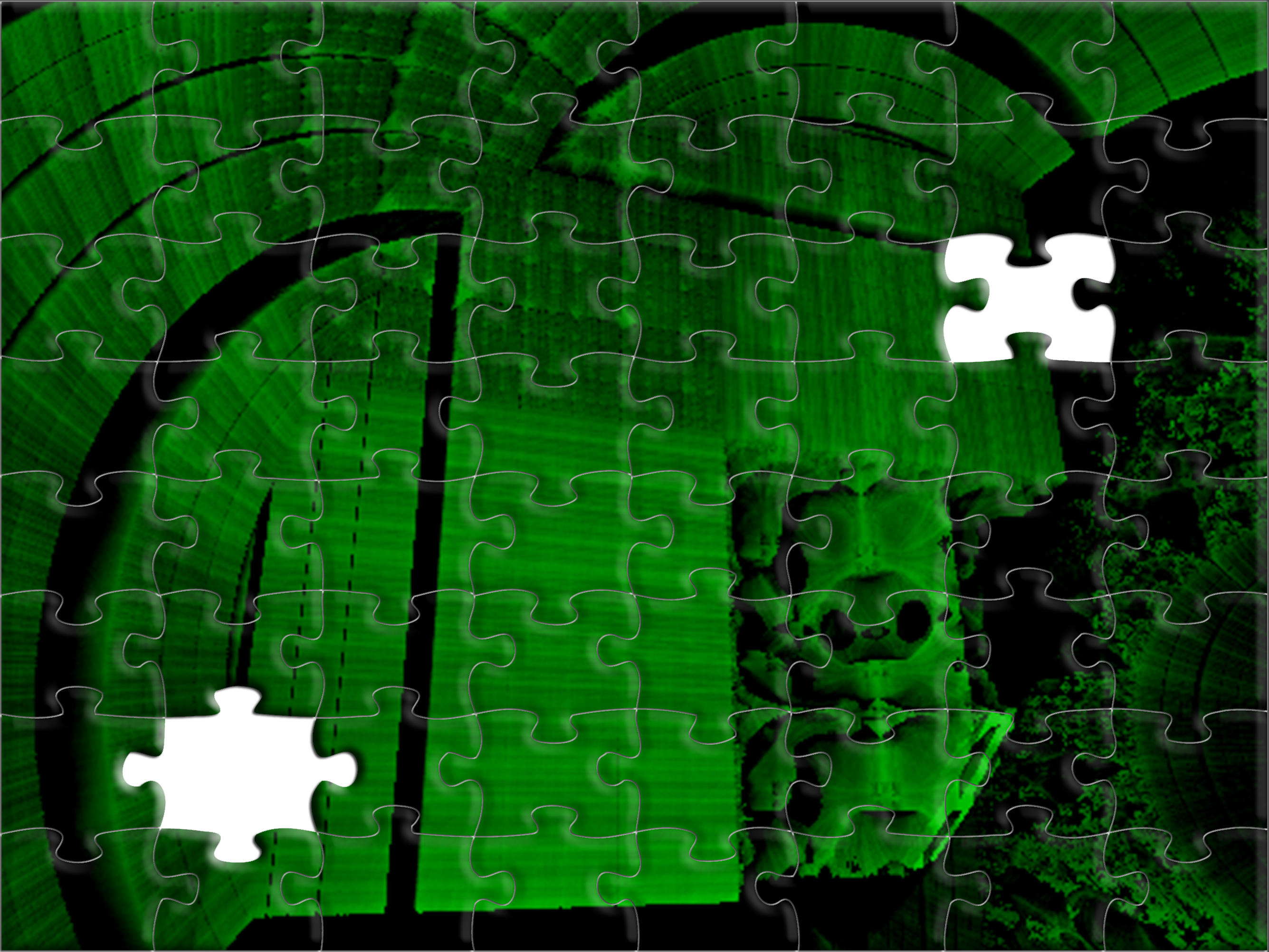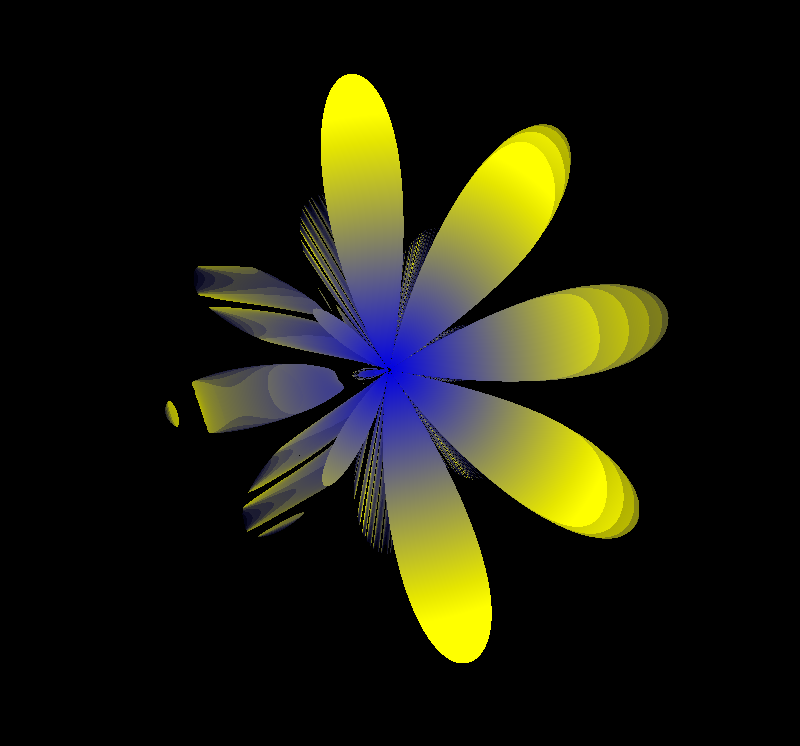Recursive:

• Uses deeper recursion to draw
finer details

• Stops at a base case

• Examples include trees and the
Cantor set

tree(length, angle, threshold, step) {
rotate(angle);
drawLine(0, -length);
translate(0, -length);
if(length > threshold) {
tree(length * step, angle, threshold, step);
tree(length * step, -angle, threshold, step);
}
}
A tree fractal. Uses recursion to define its detail.

Escape-Time (Iterative):

• Uses higher iterations to draw finer details

• Stops when a threshold distance is reached

• Calculated with complex or
hypercomplex numbers

• Examples include the Julia, Mandelbrot,
and Newton basin

mandelbulb(z, C, n, iterations, threshold) {
result = 0;
for(i=0;i<iterations;i++) {
z = pow(z, n) + C;
result++;
if(length(z) > pow(threshold, 2)) {
break;
}
}
return result;
}
A Mandelbulb changing from n=1 to n=8.

Drawing:

• Uses a graphics context to draw

• Most basic and least efficient method

• Fixed function pipeline

• Recursive fractals often use this method

draw(context) {
context.setColor(#000000);
context.fillRect(0, 0, width, height);
context.translate(140, 283);
context.rotate(16);
context.scale(4);
context.setColor(#ff0000);
context.drawLine(90, 45, 450, 900);
...
}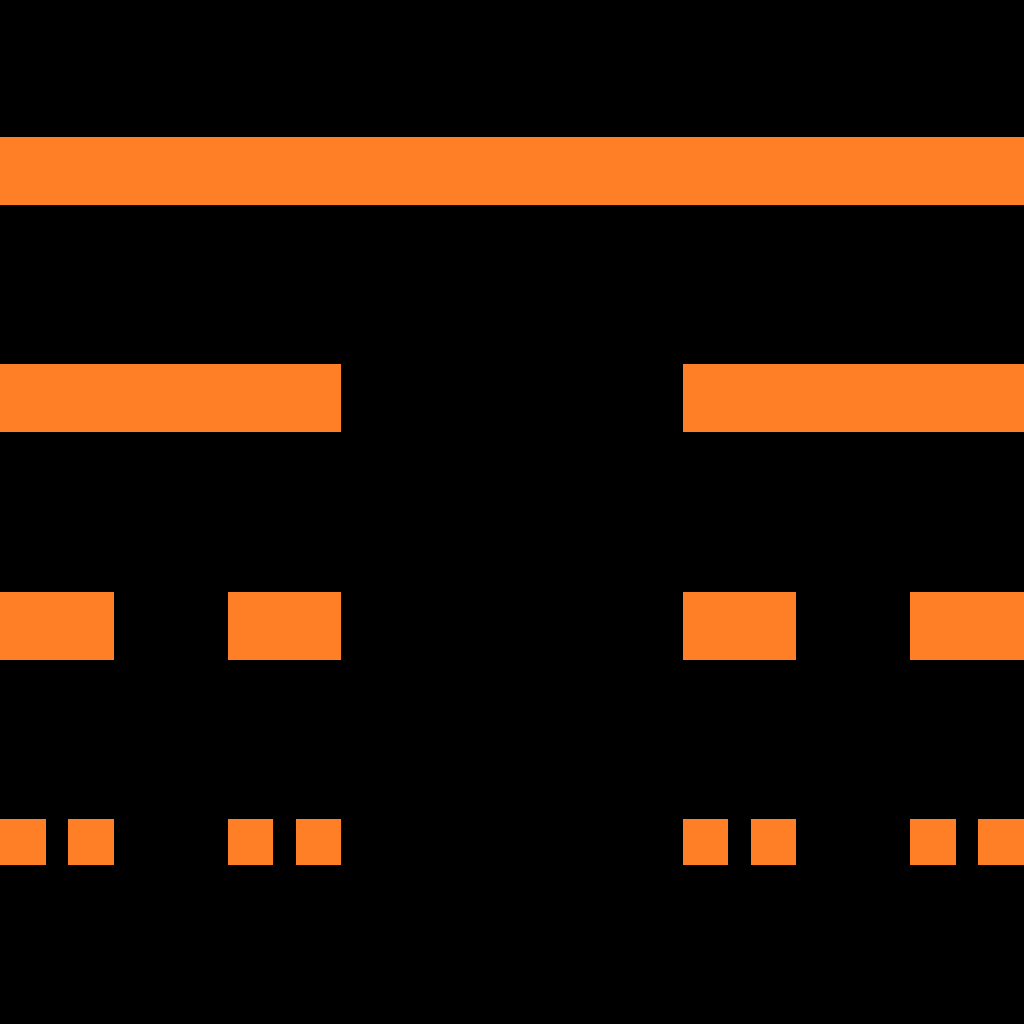The Cantor set after three iterations. Rendered using the fixed-function pipeline.

Complex Plot:

• Plots points on the complex plane

• Iterates through each pixel

• Plots the fractal function

• Can utilize hardware acceleration

complexPlot(pixel, resolution, min, max, rotation) {
point = scale(pixel, resolution, min, max);
point = rotate(point, rotation);
point = translate(point, min, max);
return f(point);
}
scale(p, resolution, min, max) {
range = max – min;
ratio = p / resolution;
aspect = resolution.x / resolution.y;
x = min.x + range.x * ratio.x * aspect;
y = min.y + range.y * ratio.y;
return vec2(x, y);
}
rotate(p, rotation) {
rc = cos(rotation);
rs = sin(rotation);
x = p.x * rc – p.y * rs;
y = p.x * rs + p.y * rc;
return vec2(x, y);
}
translate(p, min, max) {
range = max – min;
origin = min + range / 2;
x = origin.x + p.x;
y = origin.y + p.y;
return vec2(x, y);
}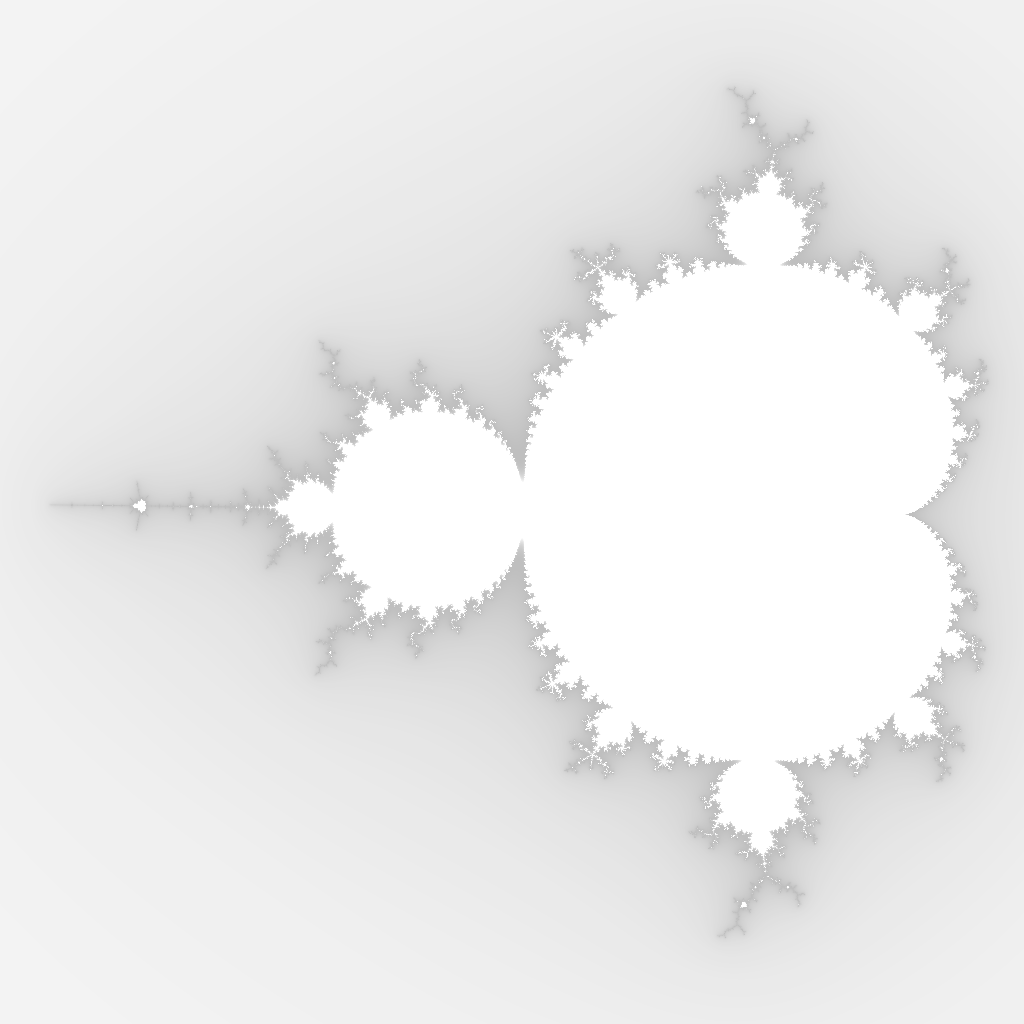Ray-Marching:

• Renders volumetric geometry
using a signed distance function

• Iterates through each pixel
on the view plane

• Rays move deeper into the scene each step

• Collisions with geometry are checked for
at each step

• Can utilize hardware acceleration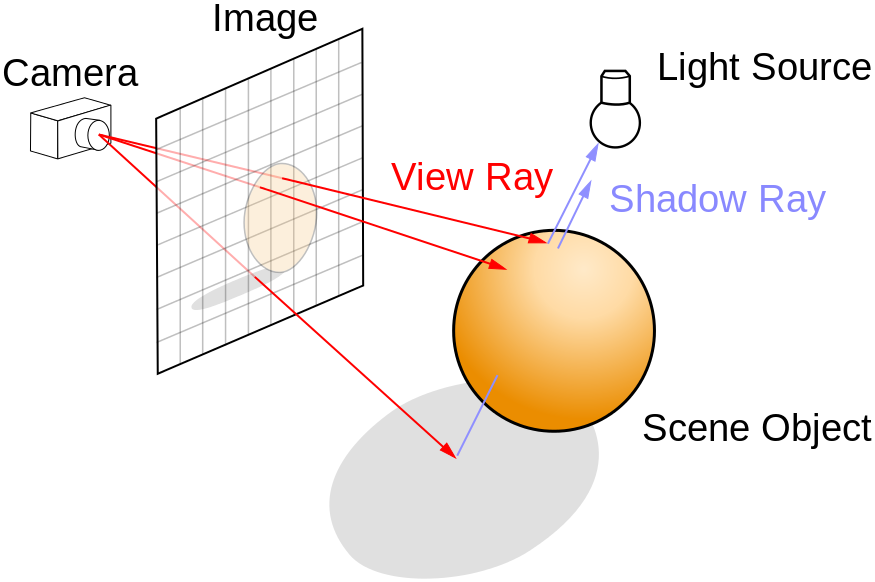Rays are emitted from the camera through each pixel to determine if the geometry in the scene is visible.
rayMarch(position, resolution, camOrigin, camLookAt,
camUp, iterations, threshold) {
result = 0;
position = scale(position, resolution);
direction = getDirection(position, camOrigin,
camLookAt, camUp);
for(i=0;i<iterations;i++) {
distance = f(position);
if(distance < threshold) {
break;
}
position += distance * direction;
result++;
}
return result / iterations;
}
scale(position, resolution) {
ratio = position / resolution;
aspect = resolution.x / resolution.y;
return vec2(ratio.x * aspect, ratio.y);
}
getDirection(position, camOrigin, camLookAt, camUp) {
right = normalize(cross(lookAt, Up));
lookAt = normalize(camLookAt);
up = normalize(camUp);
return normalize(right * position.x + up * position.y + lookAt);
}

Iterative:

• Returns a color based on values returned by
the renderer

• Many variations are possible with different
variables

• Percentage of steps required to hit the
geometry is most useful

getFragment(fragCoord, color) {
percent = f(fragCoord);
return color * percent;
}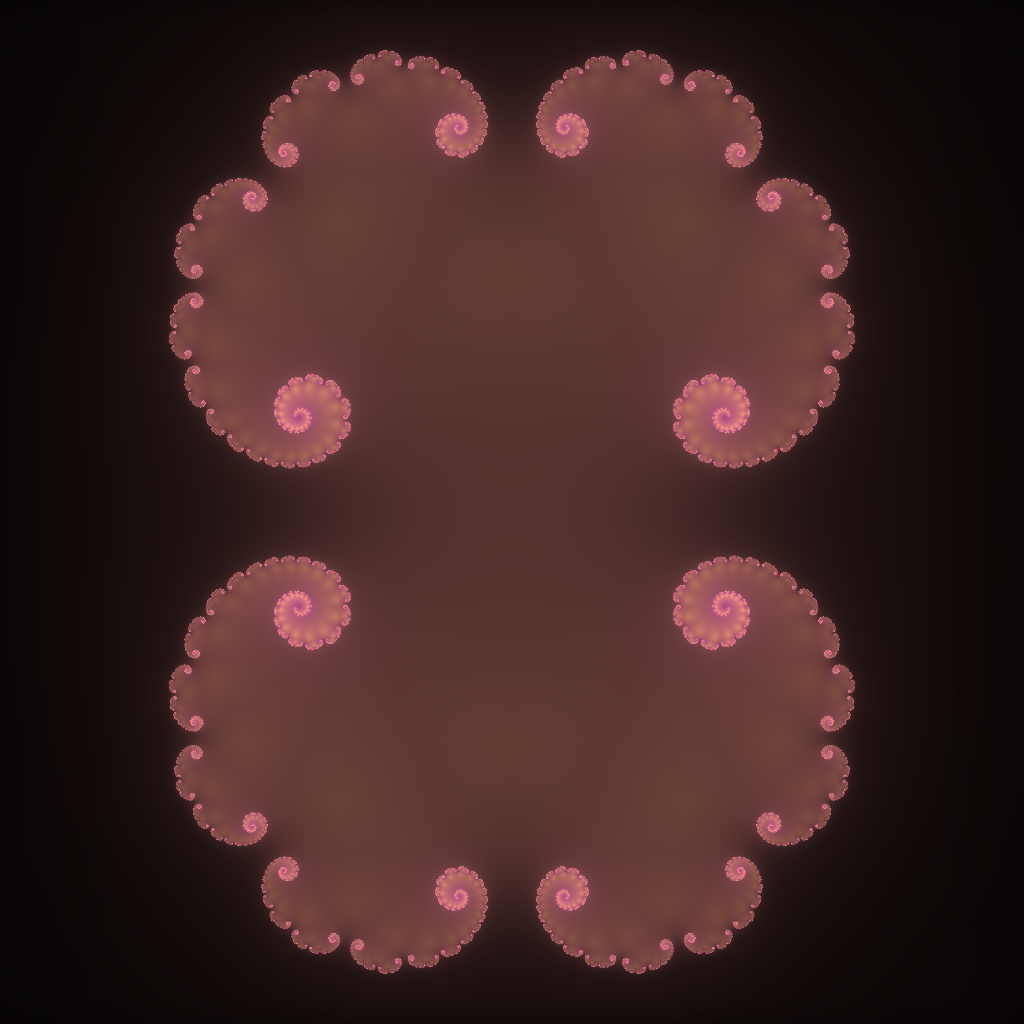Random:

• Returns a random color based on the fragment
coordinates

• Many variations are possible with different
algorithms

• Resembles television static

getFragment(fragCoord) {
float a = 12.9898;
float b = 78.233;
float c = 43758.5453;
float dt = dot(fragCoord.xy, vec2(a, b));
float sn = mod(dt, 3.14);
return fract(sin(sn) * c);
}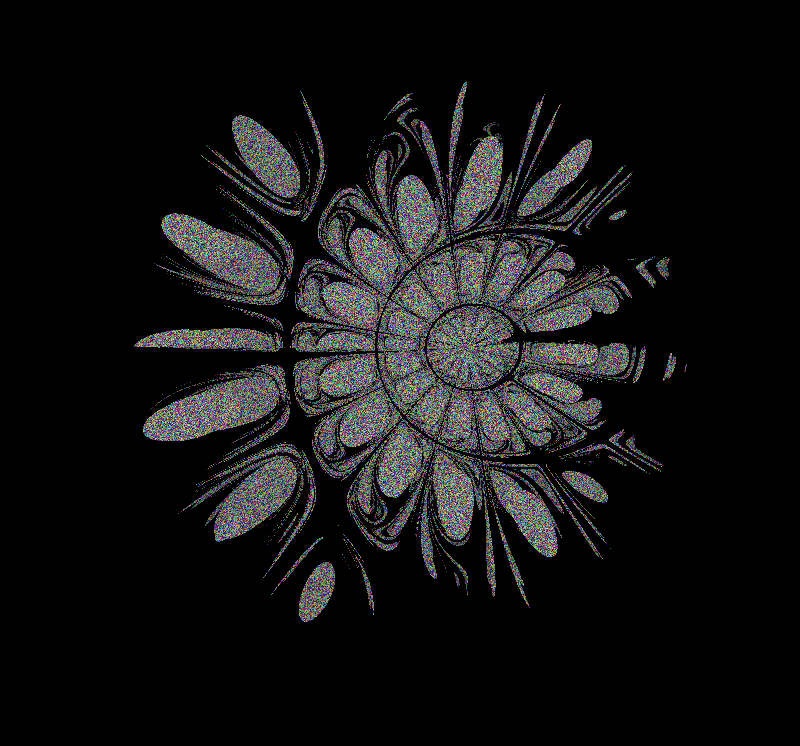A Newton knot. Shaded using a random color algorithm.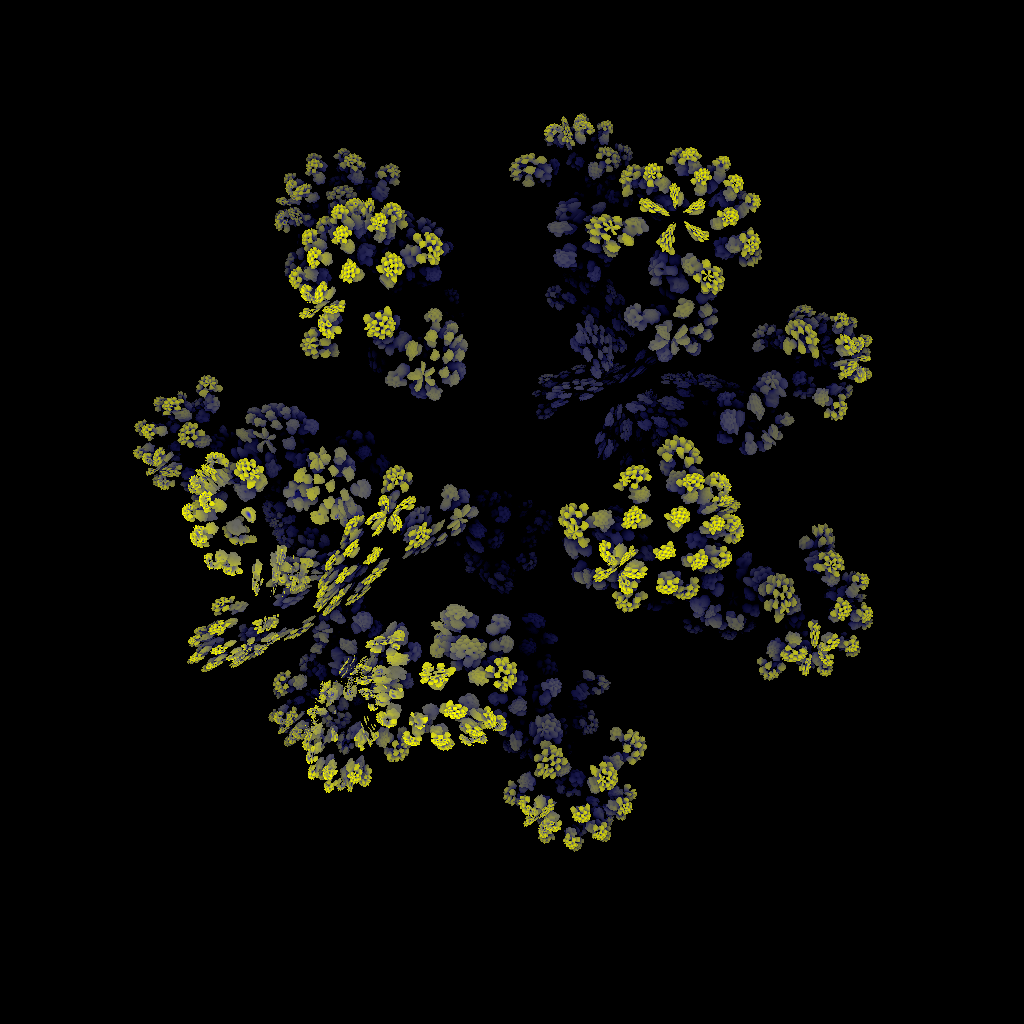The core system is:

• Portable

• An API

• Implemented in Java

The core contains:

• A document model

• A math library

• Support structures

PC Version:

• Implemented in Java Swing

• Uses JOGL for OpenGL bindings

Mobile Version:

• Implemented in Android

• Uses the Android bindings
for OpenGL ES

Design patterns:

• Observer Pattern:

Observers register with subjects and
are notified when the subject changes

• Command Pattern:

Commands separate actions from their
callers

• Interface Pattern:

Encapsulates data and responsibilities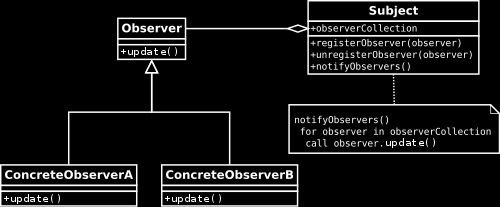The observer pattern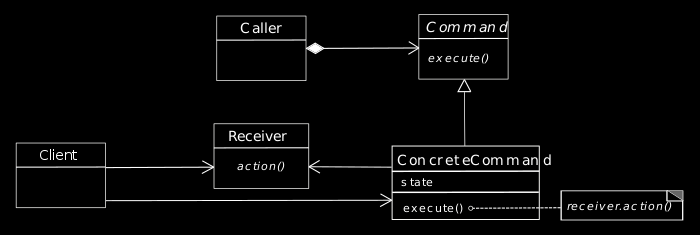The command pattern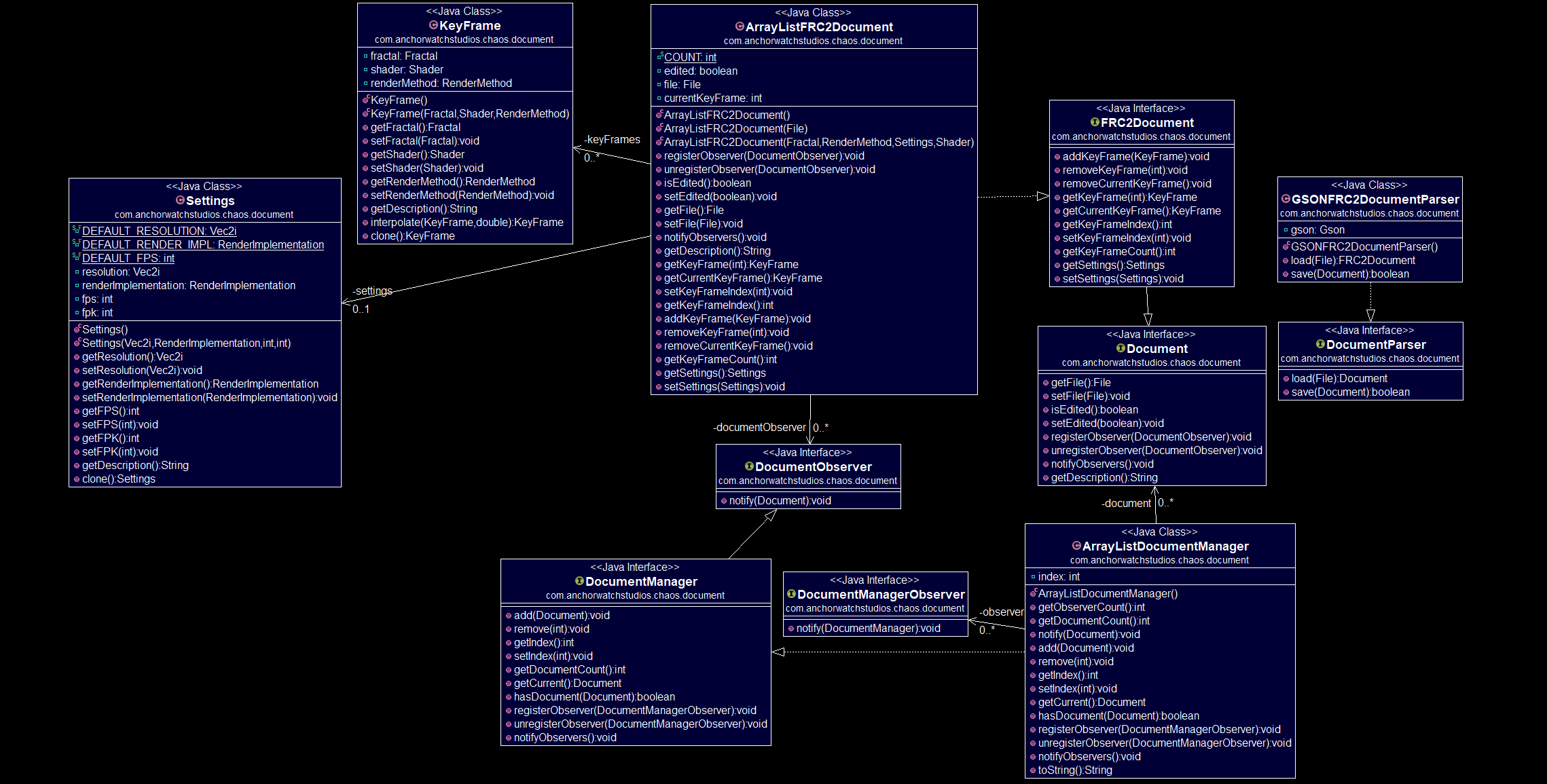The document model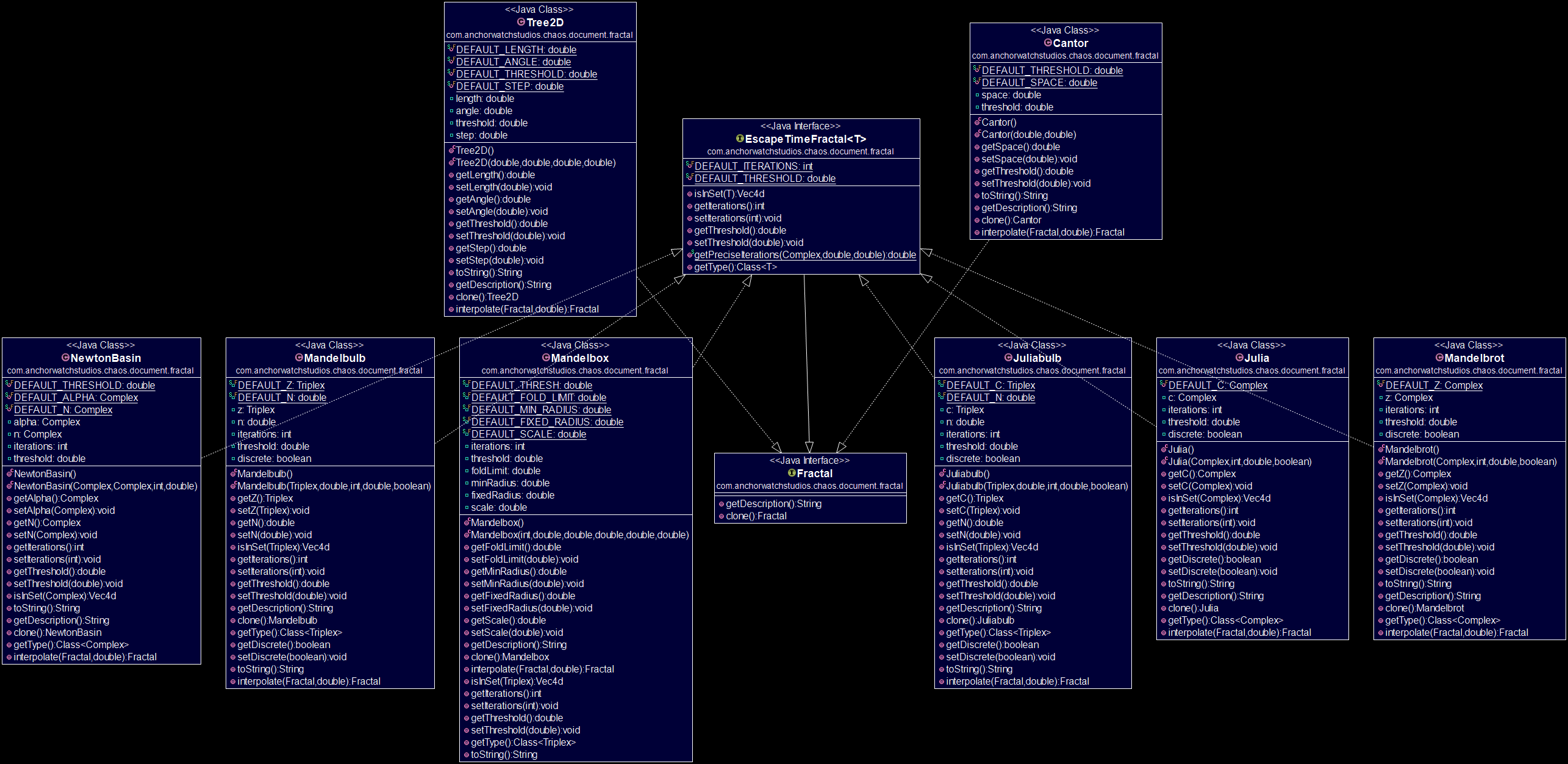The fractal model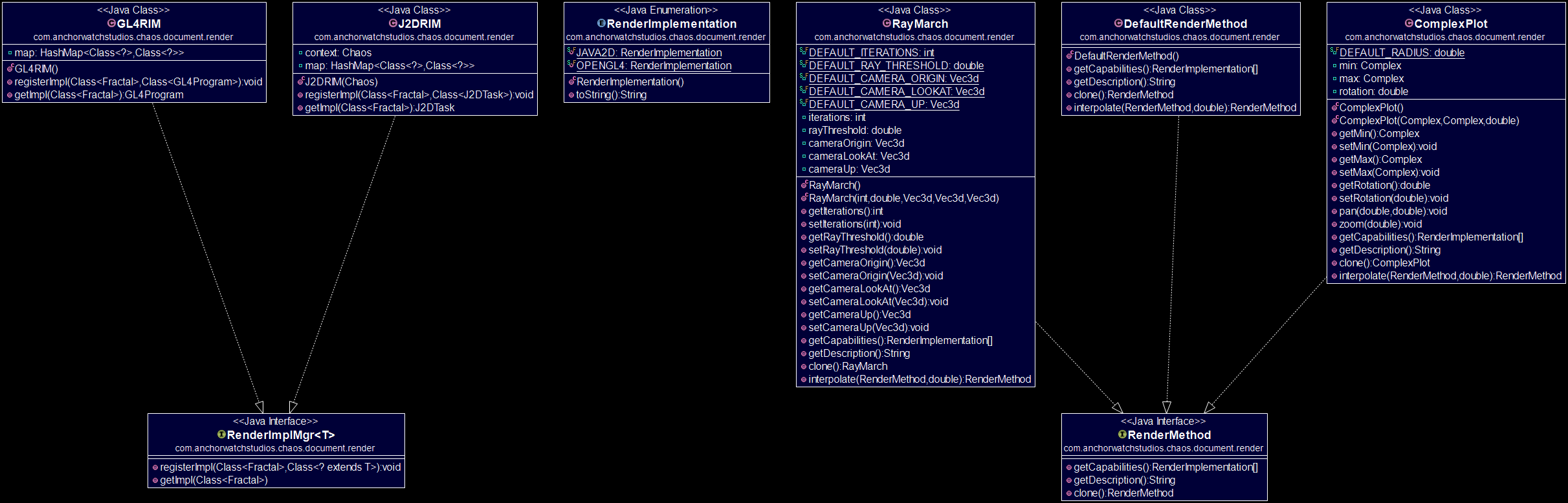The rendering model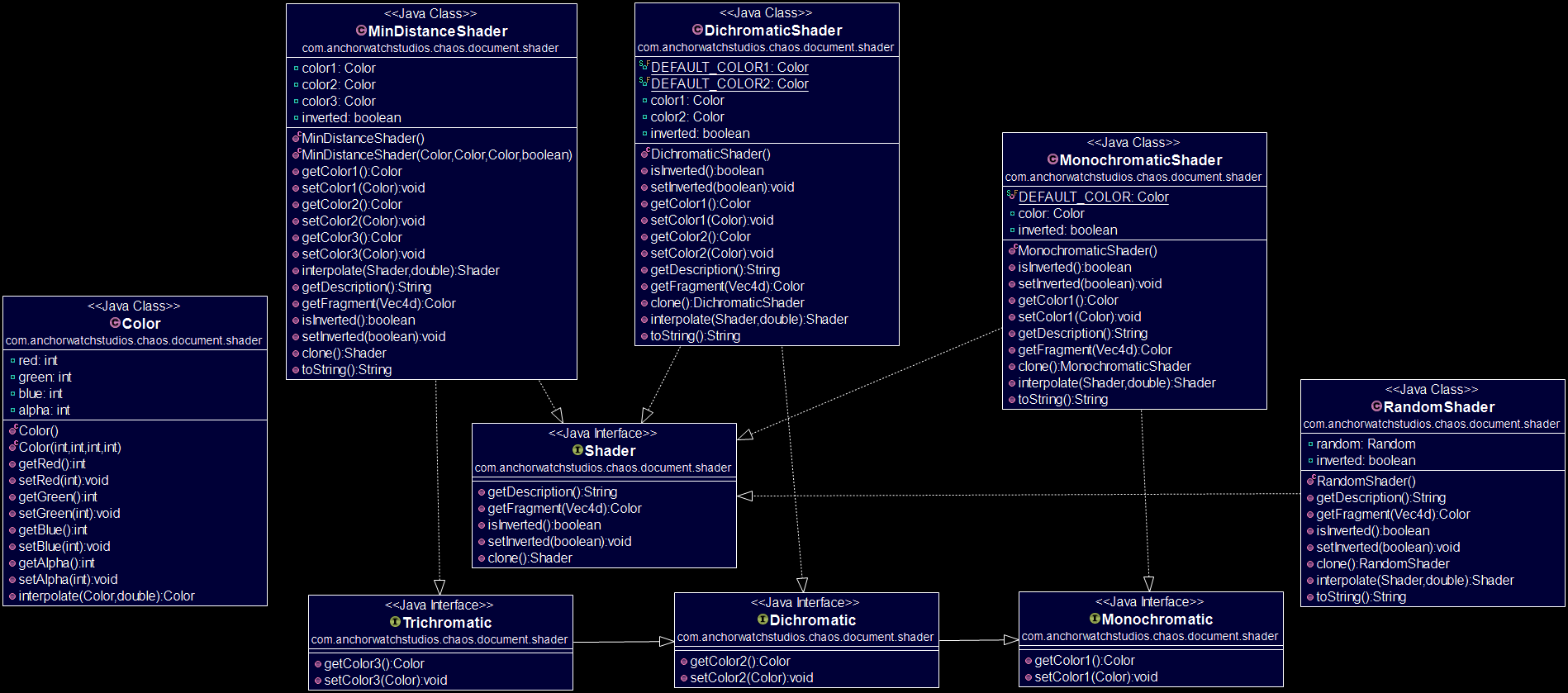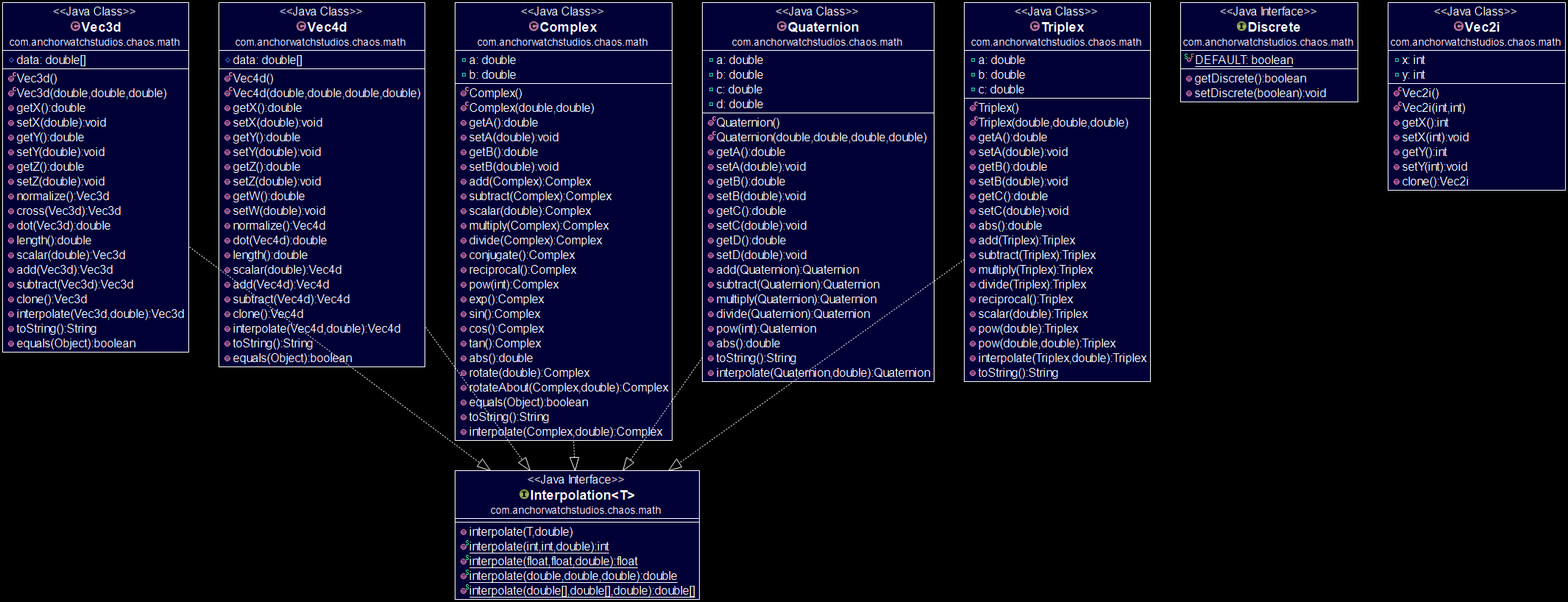The math package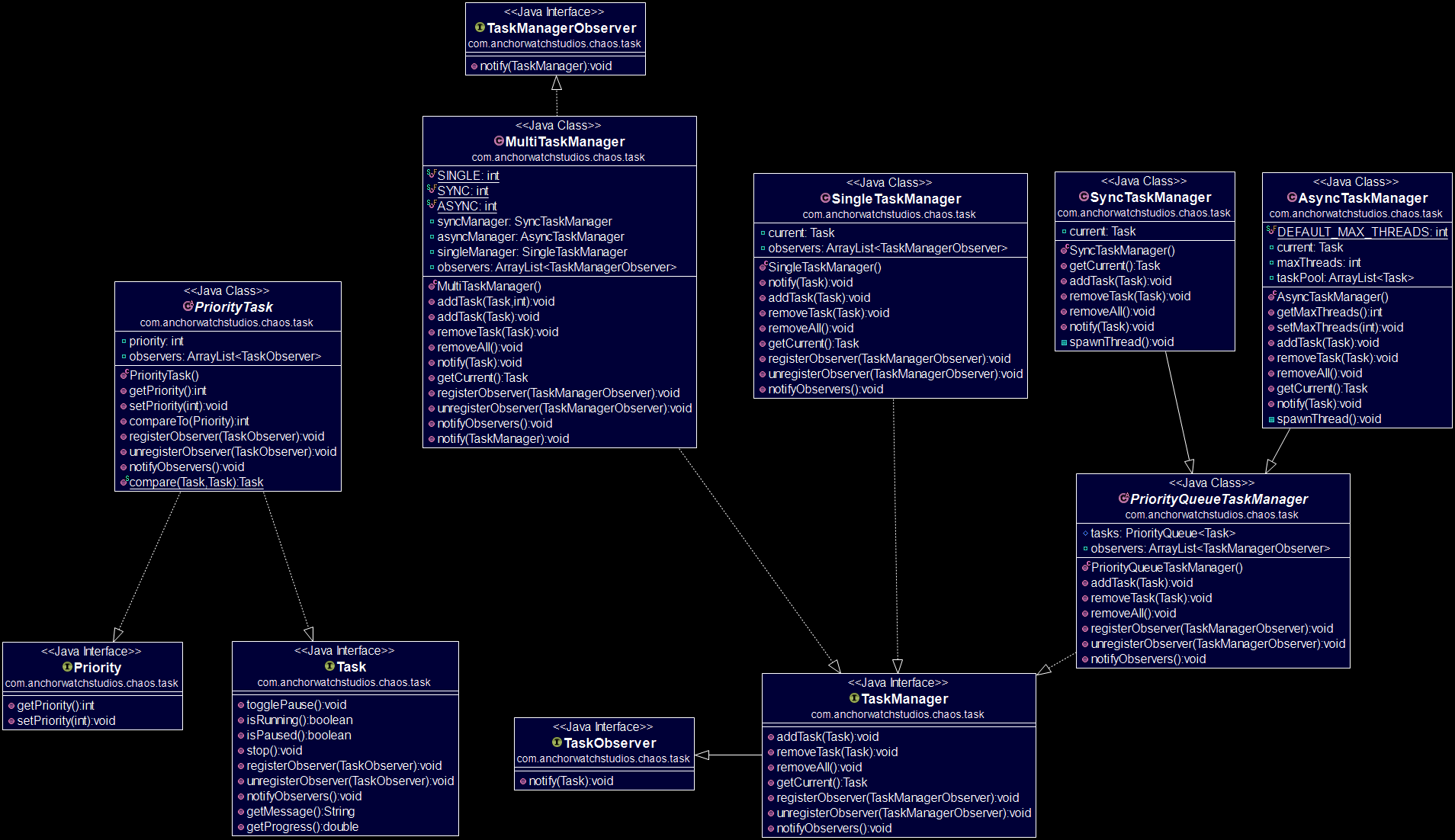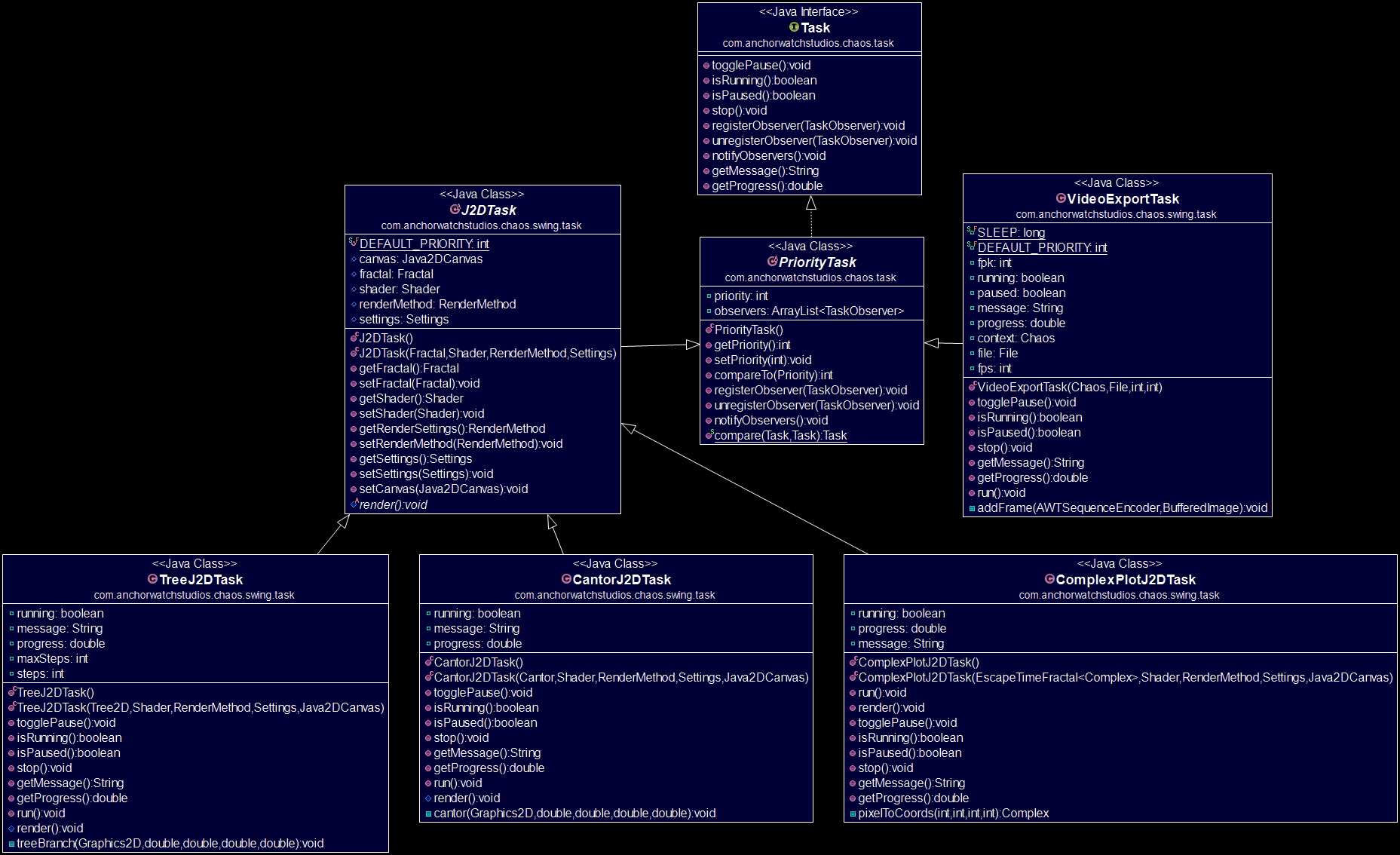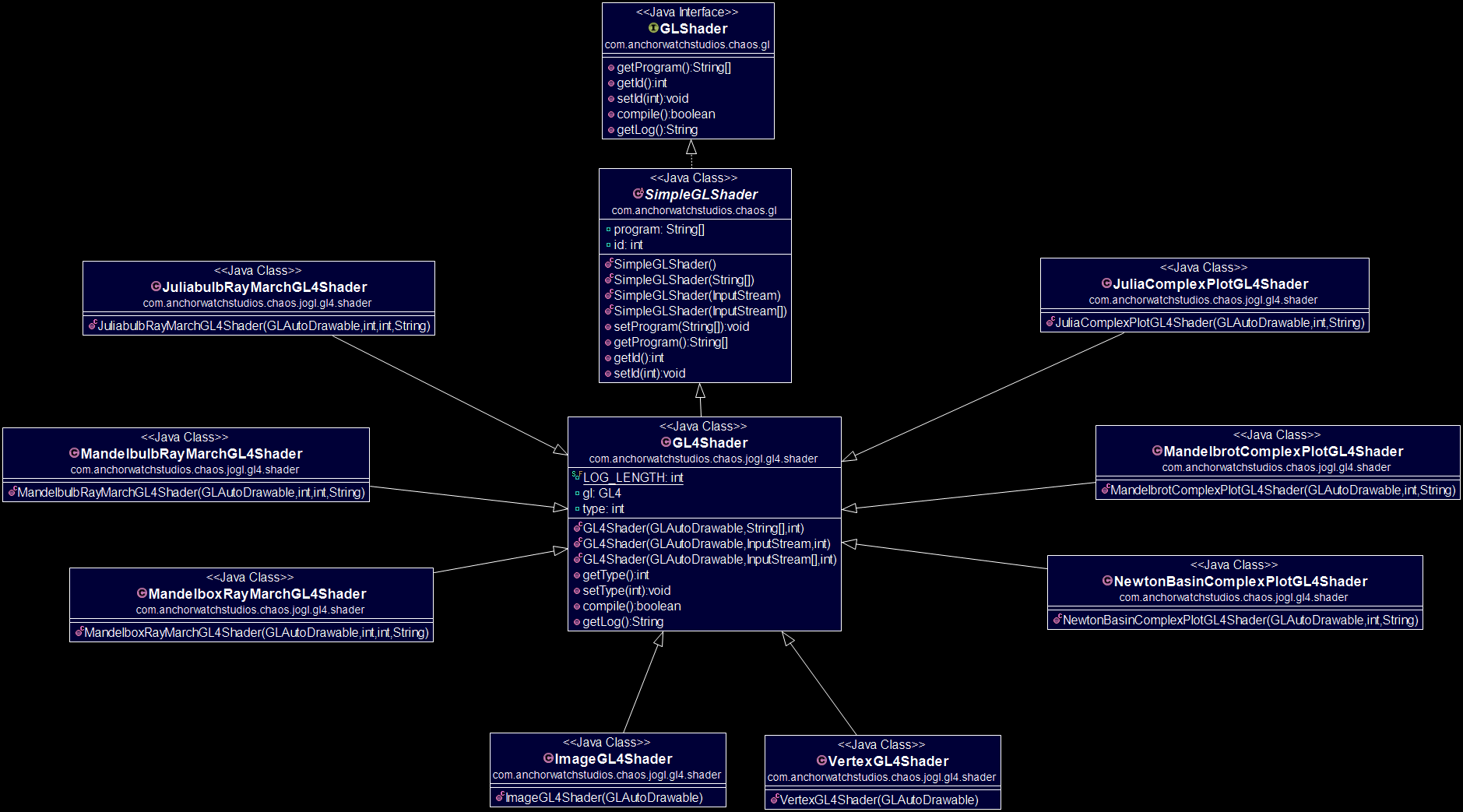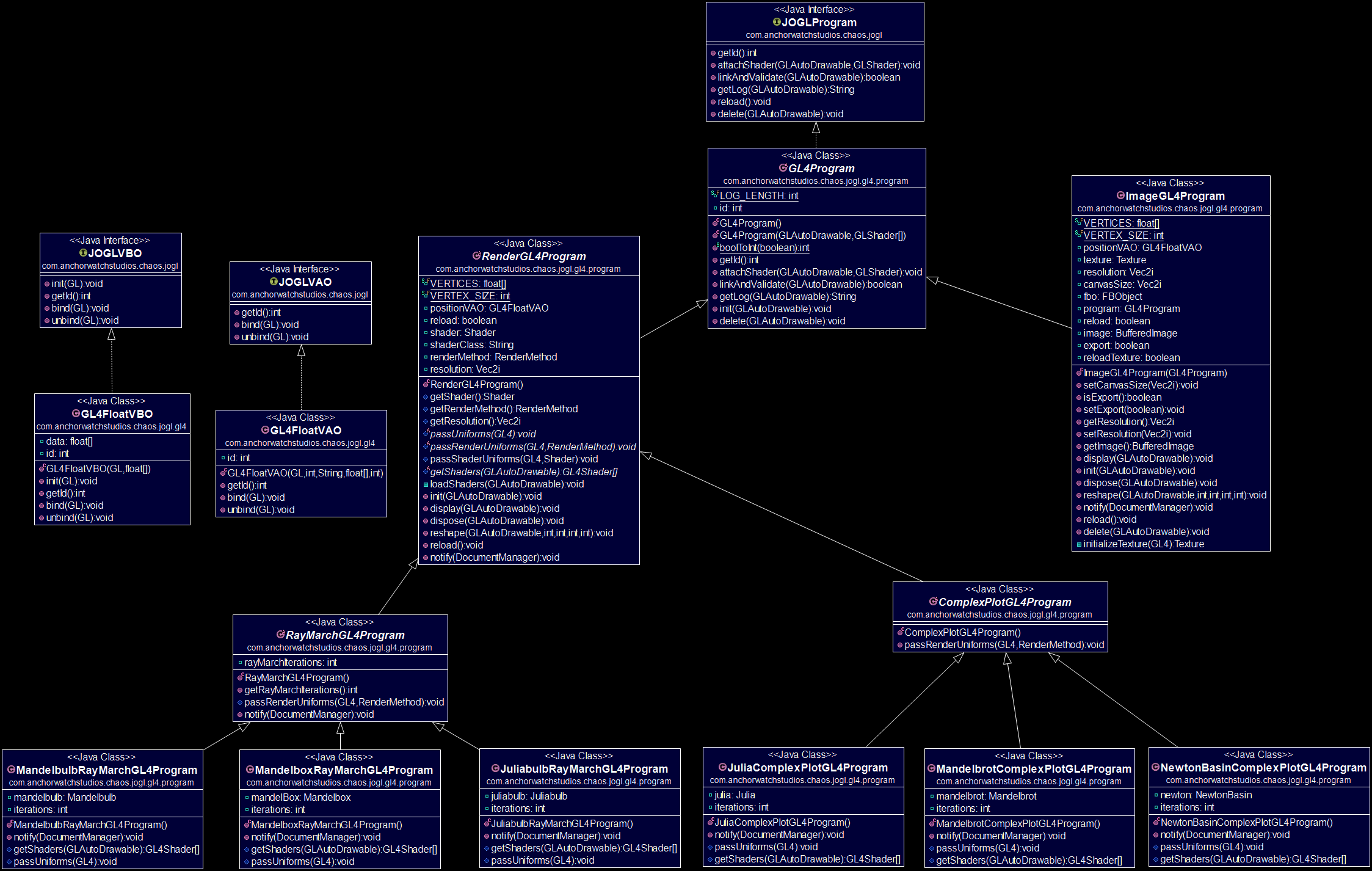The OpenGL 4.0 shader program model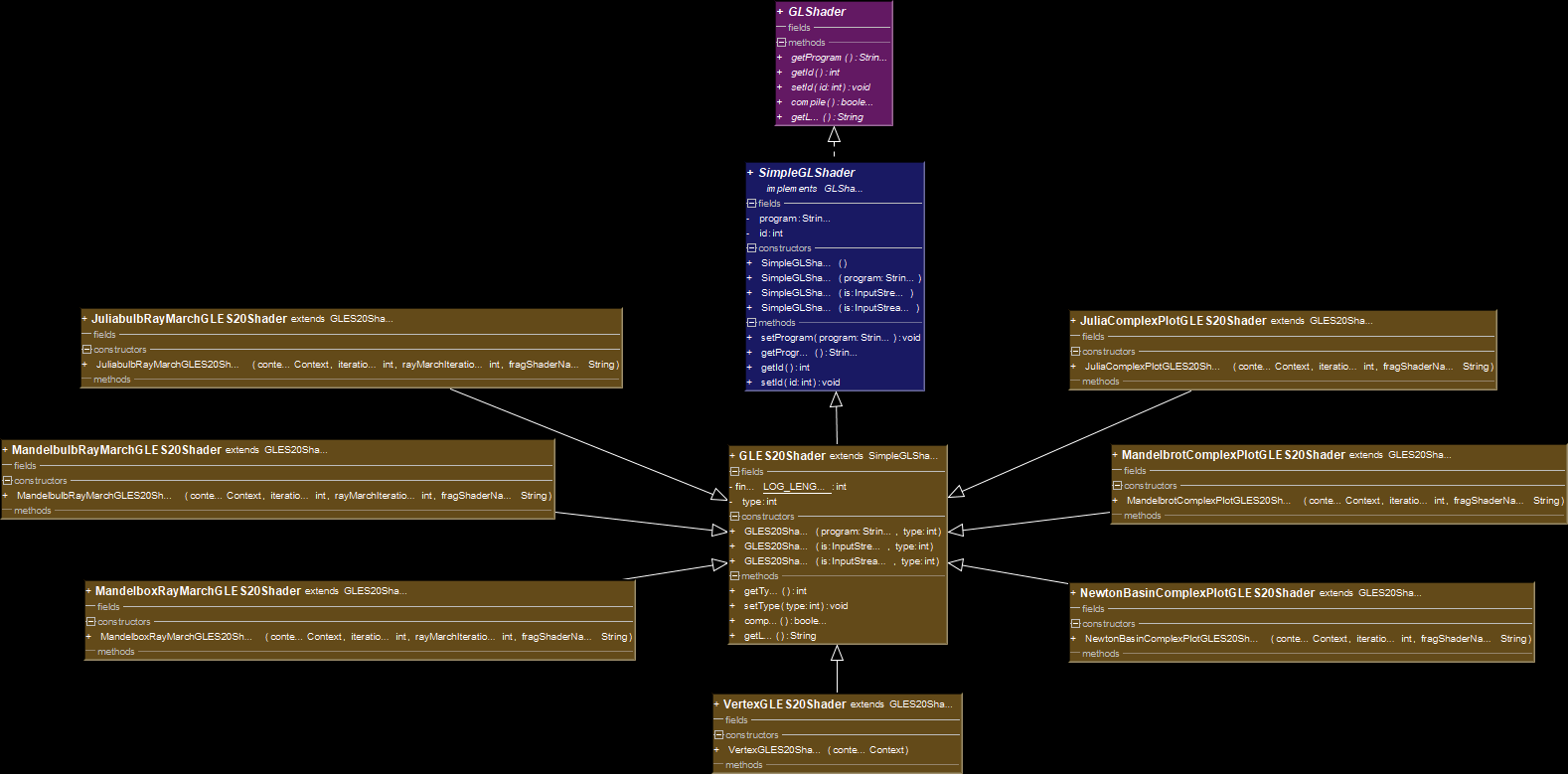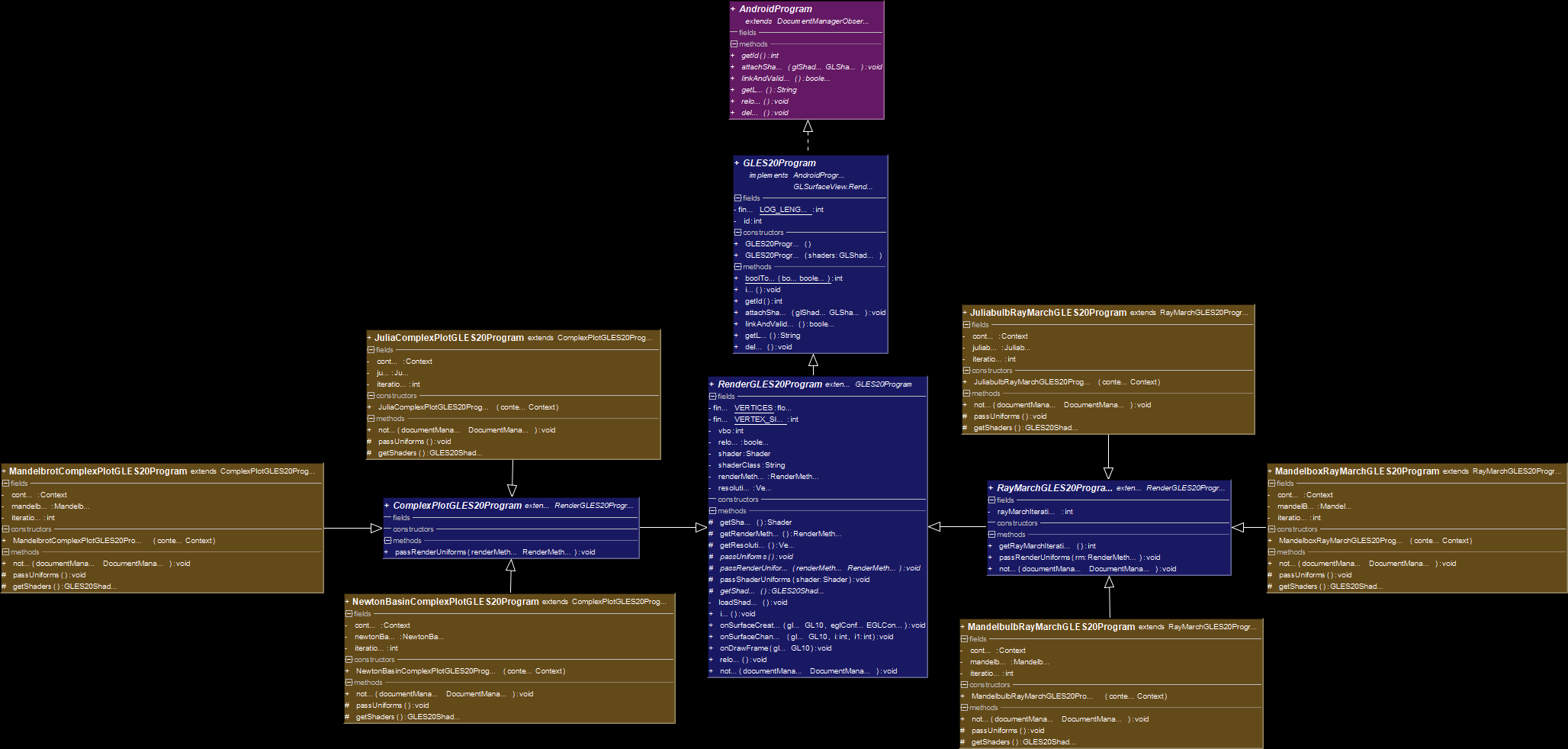The OpenGL ES shader program model

Issues Encountered:

• True resolution:

Needed to write to a framebuffer before
drawing to screen

• Observer feedback:

Observers that are also subjects update
themselves

• Document serialization:

Document version needs to be considered

• Arbitrary method signatures:

and incomplete

• Complex plot rotation:

Plot doesn't follow the mouse when aspect
ratio is not 1

• Image scaling:

Canvas image scaling is incomplete
for OpenGL 4.0

• Timeline visibility:

Timeline sometimes disappears when JPanel
is repacked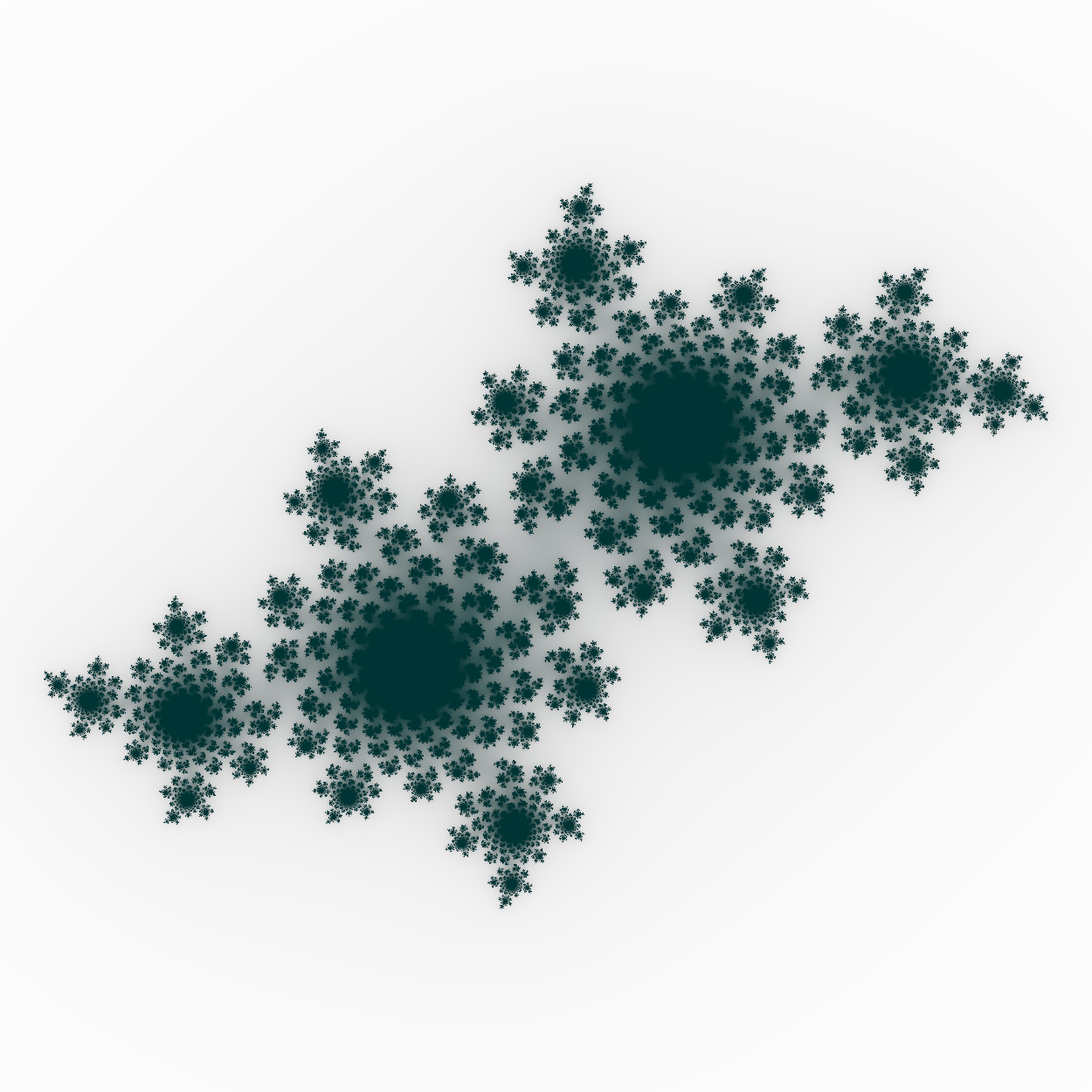• Microkernel architecture:

Creating a plugin loader will increase
modularity and extensibility

• Fractals:

and multifractals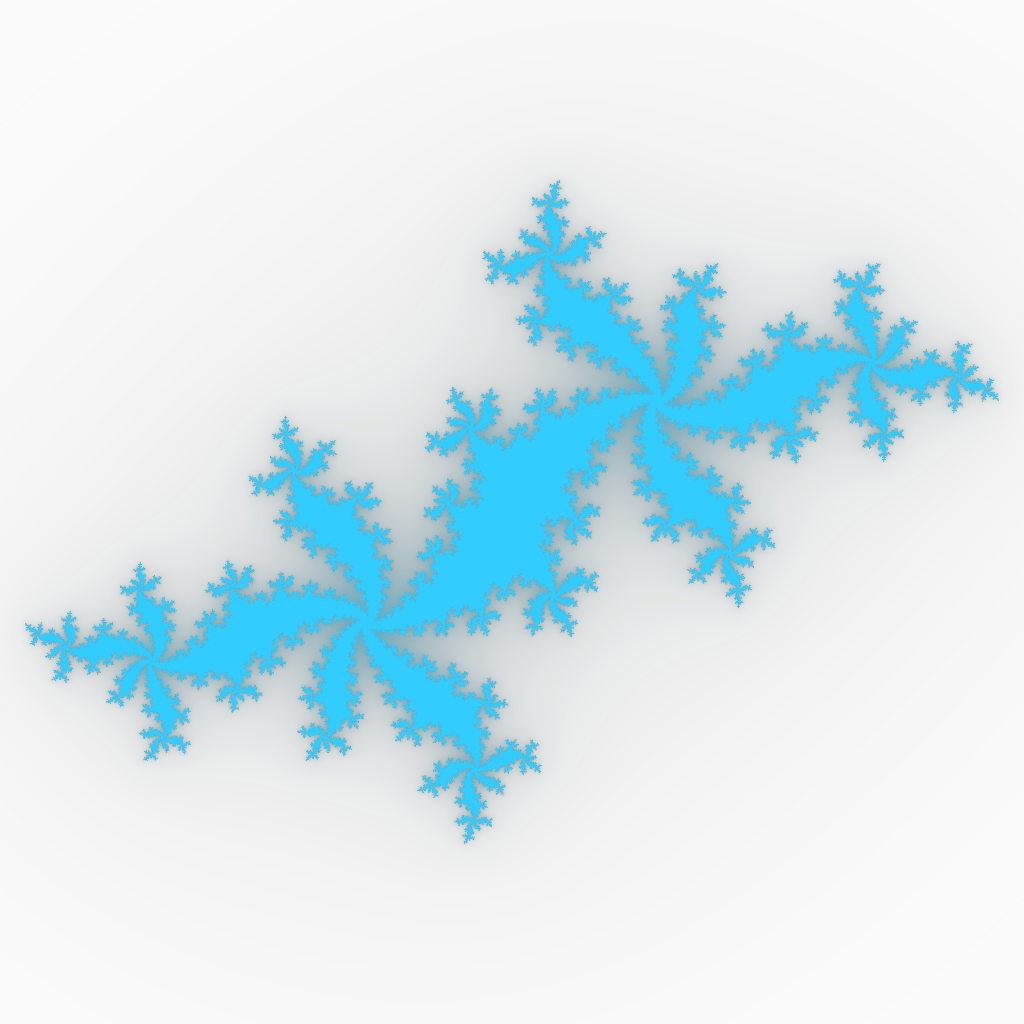Final Thoughts:

• Fractal rendering should be done in steps:

should be kept separate

• Fractals should be interfaced properly:

Fractals are very abstract and require
careful interface design

• OpenGL shaders should use an interface pattern:

Encapsulation simplifies passing uniform
variables to the shader at runtime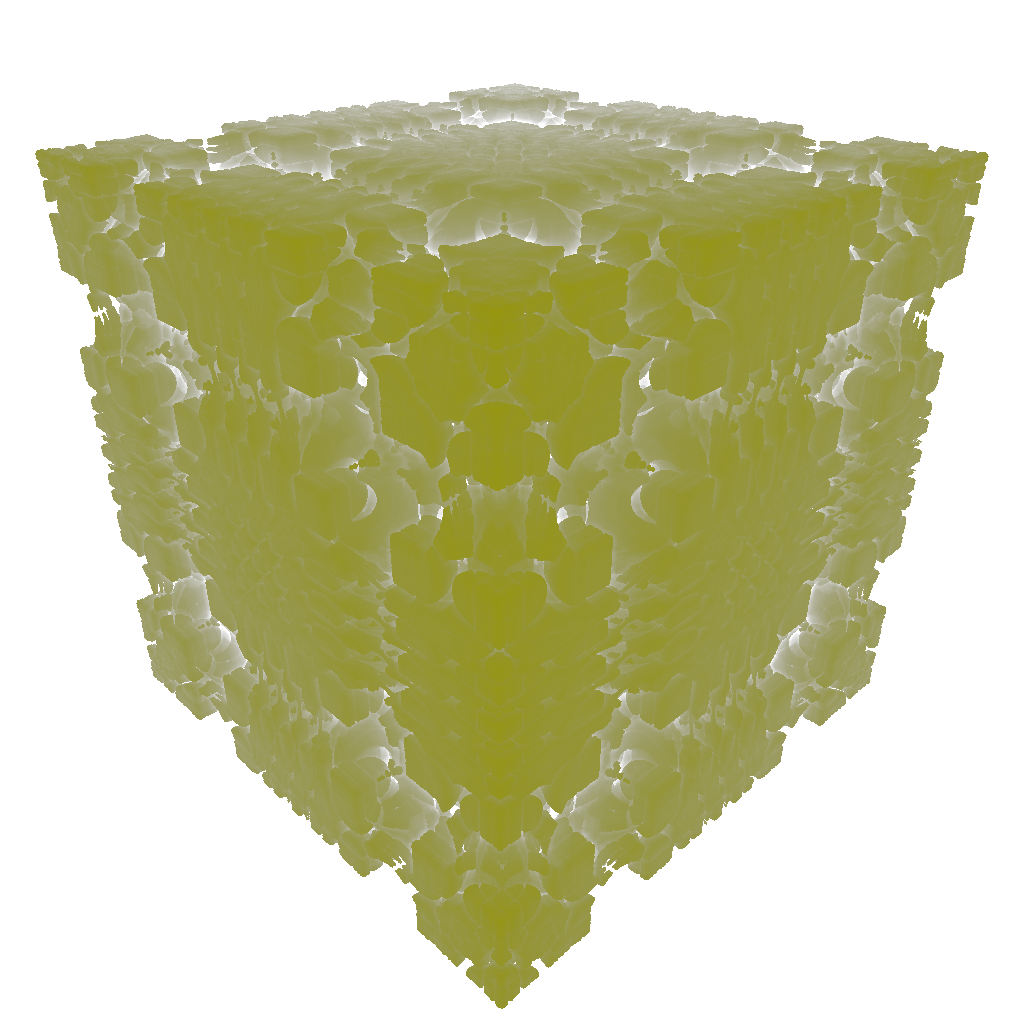Use a spacebar or arrow keys to navigate.
Press 'P' to launch speaker console.